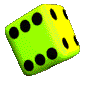# Classical Probability: Definition and Examples

Statistics Definitions > Classical Probability

Contents:

## What is Classical Probability?

Classical probability is a simple form of probability that has equal odds of something happening. For example:

• Rolling a fair die. It’s equally likely you would get a 1, 2, 3, 4, 5, or 6.
• Selecting bingo balls. Each numbered ball has an equal chance of being chosen.
• Guessing on a test. If you guessed on a multiple choice test with four possible answer A B C and D, each choice has the same odds of being picked (assuming you pick randomly and don’t follow a pattern).
• .

## Formula for Classical Probability.

The probability of a simple event happening is the number of times the event can happen, divided by the number of possible events.

The “mathy” way of writing the formula is P(A) = f / N.

P(A) means “probability of event A” (event A is whatever event you are looking for, like winning the lottery).
“f” is the frequency, or number of possible times the event could happen.
N is the number of times the event could happen.

Examples:
The odds of rolling a 2 on a fair die are one out of 6, or 1/6. That’s one possible outcome (there’s only one way to roll a 1!) divided by the number of possible outcomes (1,2,3,4,5,6).

The odds of winning Powerball are 1/292,000,000. The “1” is the number of times the event can happen (you winning), divided by the number of possible number combinations (about 292,000,000) tickets sold.

For a detailed example of using the formula, see: Probability of a Simple Event Happening.

## When You can Use the Formula.You can only use classical probability for very basic events, like dice rolls.You can only use the classical probability formula when all events are equally likely. Choosing a card from a standard deck gives you a 1/52 chance of getting a particular card, no matter what card you choose (king of hearts, queen of spades, three of diamonds etc.). On the other hand, figuring out will it rain tomorrow or not isn’t something you can figure out with this basic type of probability. There might be a 15% chance of rain (and therefore, an 85% chance of it not raining).

Dividing the number of events by the number of possible events is very simplistic, and it isn’t suited to finding probabilities for a lot of situations. For example, natural events like weights, heights, and test scores need normal distribution probability charts to calculate probabilities. In fact, most “real life” things aren’t simple events like coins, cards, or dice. You’ll need something more complicated than classical probability theory to solve them.

## Other types of probability:

• Subjective probability is based on your beliefs. For example, you might “feel” a lucky streak coming on.
• Empirical probability is based on experiments. You physically perform experiments and calculate the odds from your results.
• Axiomatic Probability: a type of probability that has a set of axioms (rules) attached to it. For example, you could have a rule that the probability must be greater than 0%, that one event must happen, and that one event cannot happen if another event happens.

## Classical Probability Examples in Real Life

Classical probability can only be applied when there are a finite number of choices that have equal probability. As such, it’s difficult to find classical probability examples in real life because most things in life do not have equal probability.

One time when you use classical probability is when you guess on a multiple choice test. Let’s say you have four choices: A, B, C, and D. Each of these options has an equal chance of being correct, which means you have a 25% chance of getting the question right.

Lottery machines—like those used for Mega Millions or Powerball— contain numbered ping pong balls that are mixed with spinning paddles or jets of air. Each of the ping pong balls has an equal probability of being chosen.

If there aren’t many classical probability examples in real life, you may be wondering what the point of learning it is. The answer is that it’s a building block for other areas of probability—like the counting rule. It also extends to more complex situations, like quantum theory, which shares many important properties with classical probability theory .

## References

 Rau, J. (2007). On quantum vs. classical probability
Lottery Machine image by: katorisi, CC BY 3.0 via Wikimedia Commons.

CITE THIS AS:
Stephanie Glen. "Classical Probability: Definition and Examples" From StatisticsHowTo.com: Elementary Statistics for the rest of us! https://www.statisticshowto.com/classical-probability-definition/